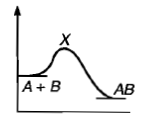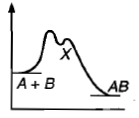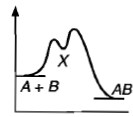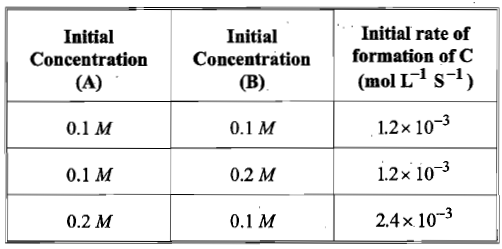# NEET and AIPMT NEET Chemistry Chemical Kinetics MCQ Questions SolvedFor a certain reaction a plot of [(c0-c)/c] against time t, yields a straight line. c0 and c are concentrations of reactant at t = 0 and t = t respectively. The rate of reaction is:

(a) 3

(b) 0

(c) 1

(d) 2

(d) For II order reaction (c0-c)/c = K.t.c0.

Difficulty Level:

• 9%
• 40%
• 34%
• 19%
Crack NEET with Online Course - Free Trial (Offer Valid Till September 18, 2019)

Hydrogenation of vegetable ghee at 250C reduces pressure of H2 from 2 atom to 1.2 atom in 50 minute. The rate of reaction in terms of molarity per second is:

(a)1.09 x 10-6

(b)1.09 x 10-5

(c)1.09 x 10-7

(d)1.09 x 10-8

(b) The change in molarity=n/V = $∆$P/RT = 0.8/(0.0821*273) = 0.0327

rate of reaction = change in molarity per sec = 0.0327/(50*60)=1.09*10-5 mil litre-1 sec-1

Difficulty Level:

• 17%
• 59%
• 20%
• 6%
Crack NEET with Online Course - Free Trial (Offer Valid Till September 18, 2019)

A hypothetical reaction, A2 +B2 $\to$ 2AB mechanism as given below;

A2 $⇌$A+ A          ............(Fast)

A+B2 → AB + B   ............(Slow)

A+ → AB         ............(Fast)

The order of the overall reaction is:

(a) 2

(b) 1

(c) 1.5

(d) zero

(c) r = k[A][B2]

and Kc = [A][A]/[A2] or [A] = k1/2c [A2]1/2

Thus, r = k .  k1/2c [A2]1/2[B2] = k'[A2]1/2[B2]

Difficulty Level:

• 28%
• 16%
• 50%
• 7%
Crack NEET with Online Course - Free Trial (Offer Valid Till September 18, 2019)

Following mechanism has been proposed for a reaction,

2A+B $\to$D+E

A+B$\to$ C+D    ...(Slow)

A+ C$\to$ E         ...(Fast)

The rate law expression for the reaction is:

(a) r = K[A]2[B]

(b) r=K[A][B]

(c) r= K[A]2

(d) r= K[A][C]

(b) The rate expression is derived for slowest step of mechanism.

Difficulty Level:

• 21%
• 68%
• 9%
• 5%
Crack NEET with Online Course - Free Trial (Offer Valid Till September 18, 2019)

In gaseous reactions important for the understanding of the upper atmosphere H2O and O react bimolecularly to form two OH radicals. $∆$H for this reaction is 72kJ at 500 K and Ea is 77 kJ mol-1, then Ea for the bimolecular recombination of two OH radicals to form H2O and O is:

(a) 3 kJ mol-1

(b) 4 kJ mol-1

(c) 5 kJ mol-1

(d) 7 kJ mol-1

(c) H2O + O $\to$2OH; $∆$H = 72KJ at 500K;

Given Ea = 77KJmol-1

2OH $\to$H2O + O ; Ea.B.R

77=72+Ea.B.R

Ea.B.R = 5 KJmol-1

Difficulty Level:

• 9%
• 21%
• 67%
• 5%
Crack NEET with Online Course - Free Trial (Offer Valid Till September 18, 2019)

For a reaction A Product, rate law is $-\frac{d\left[A\right]}{dt}=K\left[A{\right]}_{0}$. The concentration of A left after time t when $t=\frac{1}{K}$ is:

(a) $\frac{\left[A{\right]}_{0}}{e}$

(b)

(c) $\frac{\left[A{\right]}_{0}}{{e}^{2}}$

(d) $\frac{1}{\left[A{\right]}_{0}}$

(a) For I order reaction:   $\left[A{\right]}_{t}=\left[A{\right]}_{0}×{e}^{-Kt}=\left[A{\right]}_{0}{e}^{-K×\frac{1}{K}}=\frac{\left[A{\right]}_{0}}{e}$

Difficulty Level:

• 44%
• 23%
• 15%
• 20%
Crack NEET with Online Course - Free Trial (Offer Valid Till September 18, 2019)

For an exothermic chemical process occurring in two steps as;

(i) A+B$\to$X(Slow)

(ii) X$\to$AB (Fast)

The progress of the reaction can be best described by:

(a)(b)(c)(d) all of these

(a) Note that after formation of X (a slow process) the decomposition of X is fast; the energy of activation for formation of X is high.

Difficulty Level:

• 42%
• 27%
• 16%
• 17%
Crack NEET with Online Course - Free Trial (Offer Valid Till September 18, 2019)

For the non-stoichiometric reaction 2A + B $\to$ C +D, the following kinetic data were obtained in three separate experiments, all at 298 K.The rate law for the formation of C is:

(a) d[C]/dt = k[ A][B]

(b) d[C]/dt = k[ A]2[B]

(c) d[C]/dt = k[ A][B]2

(d) d[C]/dt = k[ A]

(d) Let rate expression be r = k[ A]m[B]n

1.2 x 10-3 = k(0.1)m(0.1)n             ......(i)

1.2 x 10-3 = k(0.1)m(0.2)n             ......(ii)

2.4 x 10-3 = k(0.2)m(0.1)n             ......(iii)

By (i) and (ii) n=0

By (i) and (iii) m=1

dc/dt = K[A]1

Difficulty Level:

• 15%
• 27%
• 11%
• 49%
Crack NEET with Online Course - Free Trial (Offer Valid Till September 18, 2019)

For the reaction N2 + 3H2 $\to$ 2NH3, the rate d[NH3]/dt = 2x10-4 Ms-1 .Therefore, the rate -d[N2]/dt is given as:

(a) 10-4 Ms-1

(b) 104 Ms-1

(c) 10-2 sM-1

(d) 10-4 sM-1

(a) -d[N2]/dt = 1/2d[NH3]/dt

Difficulty Level:

• 79%
• 11%
• 6%
• 6%
Crack NEET with Online Course - Free Trial (Offer Valid Till September 18, 2019)

If 'a' is the initial concentration of a substance which reacts according to zero order kinetics and K is rate constant, the time for the reaction to go to completion is:

(a) a/K

(b) 2/Ka

(c) K/a

(d) 2K/a

(a) For zero order reaction, K =x/t

If x= a (complete reactant to react);

t = a/k

Difficulty Level:

• 72%
• 10%
• 9%
• 11%
Crack NEET with Online Course - Free Trial (Offer Valid Till September 18, 2019)